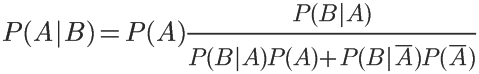# 通俗机器学习—朴素贝叶斯

1. 简介

NaïveBayes算法，又叫朴素贝叶斯算法，朴素：特征条件独立；贝叶斯：基于贝叶斯定理。属于监督学习的生成模型，实现简单，没有迭代，并有坚实的数学理论（即贝叶斯定理）作为支撑。在大量样本下会有较好的表现，不适用于输入向量的特征条件有关联的场景。

2. 基本思想

(1)病人分类的例子

某个医院早上收了六个门诊病人，如下表：

症状　　职业　　　疾病

——————————————————

P(A|B) = P(B|A) P(A) / P(B)

P(感冒|打喷嚏x建筑工人)

= P(打喷嚏x建筑工人|感冒) x P(感冒) / P(打喷嚏x建筑工人)

P(感冒|打喷嚏x建筑工人)= P(打喷嚏|感冒) x P(建筑工人|感冒) x P(感冒) / P(打喷嚏) x P(建筑工人)

P(感冒|打喷嚏x建筑工人)= 0.66 x 0.33 x 0.5 / 0.5 x 0.33= 0.66

(2)朴素贝叶斯分类器的公式

P(C|F1F2...Fn) = P(F1F2...Fn|C)P(C) / P(F1F2...Fn)

P(F1F2...Fn|C)P(C)的最大值。

P(F1F2...Fn|C)P(C) = P(F1|C)P(F2|C) ... P(Fn|C)P(C)

(3)拉普拉斯平滑（Laplace smoothing）

也就是参数为1时的贝叶斯估计，当某个分量在总样本某个分类中（观察样本库/训练集）从没出现过，会导致整个实例的计算结果为0。为了解决这个问题，使用拉普拉斯平滑/加1平滑进行处理。

eg：假设在文本分类中，有3个类，C1、C2、C3，在指定的训练样本中，某个词语F1，在各个类中观测计数分别为=0，990，10，即概率为P(F1/C1)=0，P(F1/C2)=0.99，P(F1/C3)=0.01，对这三个量使用拉普拉斯平滑的计算方法如下：

1/1003 = 0.001，991/1003=0.988，11/1003=0.011

3. 实际应用场景

文本分类

---------------------

1.  条件概率公式

设A,B是两个事件，且P(B)>0,则在事件B发生的条件下，事件A发生的条件概率（conditional probability)为：

P(A|B)=P(AB)/P(B)

1.由条件概率公式得：

P(AB)=P(A|B)P(B)=P(B|A)P(A)

上式即为乘法公式；

2.乘法公式的推广：对于任何正整数n≥2，当P(A1A2...An-1) > 0 时，有：

P(A1A2...An-1An)=P(A1)P(A2|A1)P(A3|A1A2)...P(An|A1A2...An-1)

2. 基本思想

• 条件概率又称后验概率）：事件A在另外一个事件B已经发生条件下的发生概率。条件概率表示为P(A|B)，读作“在B条件下A的概率”,。

接着，考虑一个问题：P(A|B)是在B发生的情况下A发生的可能性。

• 首先，事件B发生之前，我们对事件A的发生有一个基本的概率判断，称为A的先验概率，用P(A)表示；
• 其次，事件B发生之后，我们对事件A的发生概率重新评估，称为A的后验概率，用P(A|B)表示；
• 类似的，事件A发生之前，我们对事件B的发生有一个基本的概率判断，称为B的先验概率，用P(B)表示；
• 同样，事件A发生之后，我们对事件B的发生概率重新评估，称为B的后验概率，用P(B|A)表示。

贝叶斯定理便是基于下述贝叶斯公式：

P(A|B)=P(B|A)P(A)/P(B)

根据条件概率的定义，在事件B发生的条件下事件A发生的概率是

P(A|B)=P(A∩B)/P(B)

P(B|A)=P(A∩B)/P(A)

P(A|B)P(B)=P(A∩B)=P(B|A)P(A)

接着，上式两边同除以P(B)，若P(B)是非零的，我们便可以得到贝叶斯定理的公式表达式：

P(A|B)=P(B|A)*P(A)/P(B)

贝叶斯定理应用示例:p(A|B)=.001*.99/[.99*.001+.05*(1-.0001)]=.019

1. 如果事件组B1B2.... 满足

1.B1B2....两两互斥，即 B∩ B= i≠j ， i,j=1，2，....，且P(Bi)>0,i=1,2,....;

2.B1B2....=Ω ，则称事件组 B1,B2,...是样本空间Ω的一个划分

设 B1,B2,...是样本空间Ω的一个划分，A为任一事件，则：上式即为全概率公式（formula of total probability)

2.全概率公式的意义在于，当直接计算P(A)较为困难,而P(Bi),P(A|Bi)  (i=1,2,...)的计算较为简单时，可以利用全概率公式计算P(A)。思想就是，将事件A分解成几个小事件，通过求小事件的概率，然后相加从而求得事件A的概率，而将事件A进行分割的时候，不是直接对A进行分割，而是先找到样本空间Ω的一个个划分B1,B2,...Bn,这样事件A就被事件AB1,AB2,...ABn分解成了n部分，即A=AB1+AB2+...+ABn, 每一Bi发生都可能导致A发生相应的概率是P(A|Bi)，由加法公式得

P(A)=P(AB1)+P(AB2)+....+P(ABn)

=P(A|B1)P(B1)+P(A|B2)P(B2)+...+P(A|Bn)P(PBn)

3.实例：某车间用甲、乙、丙三台机床进行生产，各台机床次品率分别为5%，4%，2%，它们各自的产品分别占总量的25%，35%，40%，将它们的产品混在一起，求任取一个产品是次品的概率。

解：设.....     P(A)=25%*5%+4%*35%+2%*40%=0.0345

4.简单举例

一个村子与三个小偷，小偷偷村子的事件两两互斥，求村子被偷的概率。

P(B)=P(A1B)+P(A2B)+P(A3B)

P(B)=P(A1)P(B|A1)+P(A2)P(B|A2)+P(A3)P(B|A3) （*）

PS: P(Ai),P(B|Ai)是已知的

P(B)=P(B)P(A1|B)+P(B)P(A1|B)+P(B)P(A1|B)的冲动？

posted @ 2018-11-17 23:55  zzlp  阅读(271)  评论(0编辑  收藏  举报